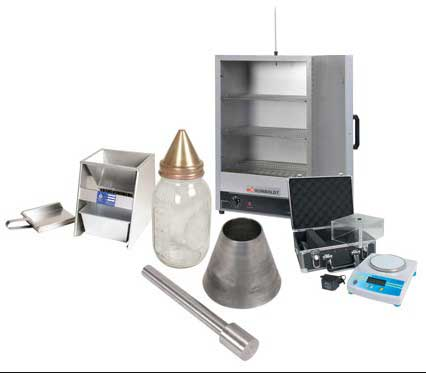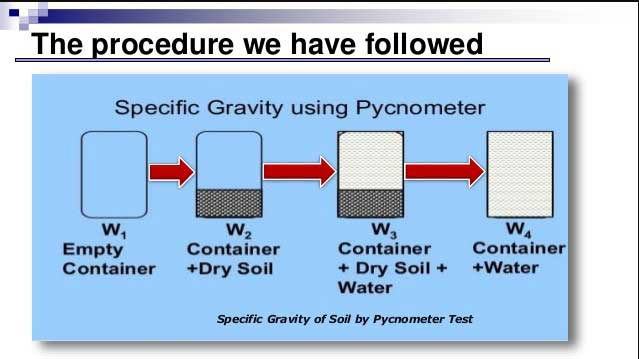# Specific Gravity of Soil Test by Pycnometer Method.

In this article, you’ll learn in details the Determination of Specific Gravity of Soil by Pycnometer; its apparatus, procedure, result, etc.

So, Let’s Get Started.

## Determination of Specific Gravity of Soil Test by Pycnometer.

For determination of specific gravity of soil by Pycnometer’

Volumes of soil solids and water are taken same.

The volume of known weight of soil grains can be obtained by using a container of known volume;

and the Archimedes Principle, “that a body submerged in a volume of water will displace a volume of water equal to the volume of the submerged body.”

The container of known volume as the volumetric flask or Pycnometer, which holds a standard volume of distilled water at 20ºC.

At temperature more than 20ºC, the volume will be slightly more, below 20ºC, the volume will be slightly less.

In routine work, generally, tap water is used instead of distilled water.

### Specific Gravity of Soil Definition.

The specific gravity of soil is defined as the unit weight of the soil mass divided by the unit weight of distilled water at 4°C.

It is some times required to compare the density of the soil solids to the density of water.

This comparison is in the form of ratio and is termed as the specific gravity of the soil.

Together with the soil moisture content and unit weight, specific gravity is frequently used to solve for the various phase relationships, such as void ratio, porosity, and degree of saturation.

Specific gravity is also required in the calculations associated with the grain size analysis, the consolidation, and compaction (Standard Proctor Test and Modified Proctor Test).

### Designation:

1. ASTM D854.
2. AASHTO T100.
3. BS 1377.

### Apparatus.

1. Volumetric Flask (250 or 500 ml) Pycnometer.

2. Vacuum Pump.

3. Mortar and Pestle.

4. Balance weighing 0.01 gm.

5. Thermometer.### Procedure.

1. Weigh the empty and dry volumetric flask / Pycnometer to the nearest 0.01 gram and record the weight as W1.

2. Take about 100 grams of oven dried soil and put it into the Pycnometer.

3. Now weigh the Pycnometer and dry soil to the nearest 0.01 gram and record the weight as W2.

4. Add water in the Pycnometer until about it is two thirds full. Gently and carefully agitate the mixture.

5. Add some more water in the Pycnometer until the bottom of the meniscus is exactly at the volume mark. Weigh the Pycnometer and record it as W3.

6. Empty the pycnometer and wash it. Then fill it with water up to the mark and weigh as W4.

7. Repeat the above Procedure three times.

8. Record the temperature of soil water mixture by the thermometer.

9. Specific Gravity of Soil is then, computed by diving the weight of soil by the weight of an equal volume of water as below.

Watch The Video Below for Better Understanding.

### Observations and Calculations.

Note:
1. wt. = weight.
2. gm = gram.
3. Gs = Specific Gravity of Soil.

Test No.123
Volume of Pycnometer (ml).500
Wt. of empty Pycnometer (W1) gm.450.47
Wt. of Pycnometer + Soil (W2) gm.950
Wt. of Pycnometer + Soil + Water (W3) gm.1850
Wt. of Pycnometer + Water (W4) gm.1600
Gs = a[(W2-W1) / (W4-W1) - ( W3-W2)]2

Typical values of correction factor “a”.

Temperature (°C)Correction Factor "a".
41.00
150.9999
200.9982
250.9971
300.9957
350.9941### Result.

Average Specific Gravity of Soil = ______________.

### Typical values of Gs.

These values can be used as a guide in determining whether the tests result are correct. The typical values of Gs as follow:

Type of SoilGs Values.
Sand.2.65 - 2.67
Silty Sand.2.67 -2.70
Inorganic Clay.2.70 - 2.80
Soil with mica or iron.2.75 - 3.00
Organic Soil.Variable but may be under 2.00

### Precautions.

1. Weigh the Pycnometer carefully.

2. Shake the Pycnometer well to extract air by fully saturating the soil.

3. Do not shake vigorously.

### Q & A about Specific Gravity of Soil.

Q.1: Define the Specific Gravity.

Ans: The unit weight of any material divided by the unit weight of distilled water.

Q.2: Why unit weight of water is taken at 4ºC.

Ans: Because at 4ºC the unit weight of water is 1.

Q.3: Give the formula to determine the specific gravity (Gs).

Ans: Gs = [(W2-W1) / (W4-W1) – ( W3-W2)].

Q.4: Give the formula to determine the corrected value of Gs.

Ans: Corrected Gs = a[(W2-W1) / (W4-W1) – ( W3-W2)].

Read Also: Determination of Field Density Test of Soil by Core Cutter Method.

1.